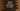# Python program to print the odd numbers in a given range## Python program to print the odd numbers in a given range :

In this tutorial, we will learn how to print odd numbers within a given range. The program will ask the user to enter the lower and upper limit of the range. It will then find out all the odd numbers in that range and print them out.

This program will use one loop to run within the user provided range. With this program, you will get a better understanding of for loop in python.

Let me show you the python program first :

### Python program using a for loop :

``````#1
lower_limit = int(input("Enter the lower limit : "))
upper_limit = int(input("Enter the upper limit : "))

#2
for i in range(lower_limit,upper_limit + 1):
#3
if(i%2 != 0):
print("{} ".format(i))``````

### Explanation :

The commented numbers in the above program denote the step numbers below :

1. Ask the user to enter the lower limit. Read it and convert it to an int. Then store it in the lowerlimit_ variable. Similarly, read the upper limit and store it in the upperlimit_ variable.
2. Use one loop. Using this loop, run between the lower limit and the upper limit that the user has entered.
3. For each value, check if it is divisible by 2 or not. If not, it is an odd number. Print out the result.

### Sample Output :

``````Enter the lower limit : 3
Enter the upper limit : 13
3
5
7
9
11
13``````

### Using a while loop :

``````lower_limit = int(input("Enter the lower limit : "))
upper_limit = int(input("Enter the upper limit : "))

while(lower_limit < upper_limit + 1):
if(lower_limit % 2 != 0):
print(lower_limit)
lower_limit += 1``````

### Explanation :

We can also solve this problem using a while loop. In this example, the while loop will run until lowerlimit_ is less than upperlimit + 1. Inside the loop, we are incrementing the value by 1 on each iteration. Using an _if condition, we are checking if the value is odd or not and printing out the odd values.

It will produce output similar to the above for loop example.

The above programs are available here on Github.

### Conclusion :

Using a loop, you can scan within a range. In this tutorial, we have learnt to solve this problem by using a for loop and a while loop. Try to run the above examples and drop one comment below if you have any queries.